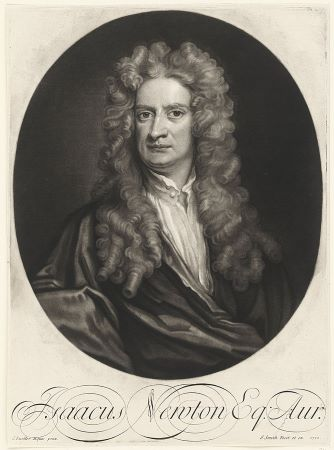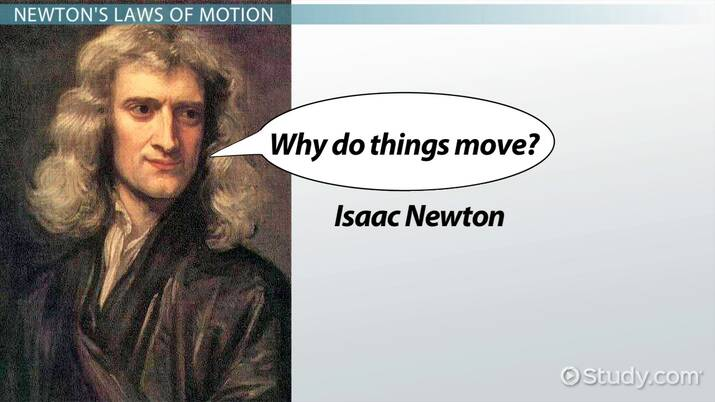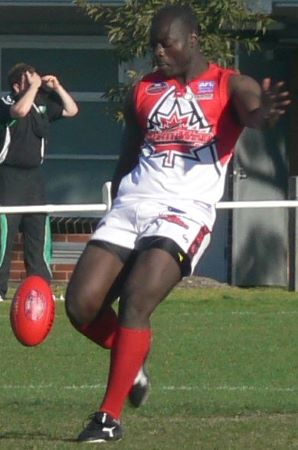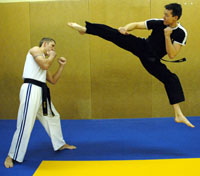# Newton's Laws of Motion

Tanya Hausman, David Wood
• Author
Tanya Hausman

Tanya has taught for 21 years, anywhere from 1st through 9th grades, as well as STEM. She has a bachelor's in elementary education with a middle school math endorsement from Oklahoma Wesleyan University. She has a current professional teaching license and years of experience creating interesting, engaging lessons for her students.

• Instructor
David Wood

David has taught Honors Physics, AP Physics, IB Physics and general science courses. He has a Masters in Education, and a Bachelors in Physics.

Learn about Sir Isaac Newton's laws of motion. Get an overview of each of Newton's three laws, see how they are related to each other, and get examples of each. Updated: 02/15/2022

Show

## Newton's Laws of MotionSir Isaac Newton was an English physicist and mathematician who lived in the 1600s and 1700s. One of his most famous works is his three laws of motion. Newton's three laws were first introduced in his book The Principia, which was published in 1687. Newton's three laws explain how objects behave when still, when moving, and how forces relate to their movement. Force is a push or pull on an object. A person can see force when a ball is kicked; how that ball moves is explained by Newton's laws. It can also be seen when NASA launches a rocket or a child makes a toy rocket. The amount of force needed to move that rocket and how it moves is explained by Newton's laws.

## Newton's Laws of Motion

The study of kinematics (1D and 2D motion) was about describing how things move, from displacements to velocity to acceleration. However, when Newton began to do his work, he was concerned with explaining why things move the way they do. He achieved that by defining his three laws of motion. These use the concept of force (a push or pull) to explain the motion we observe in the world around us. Let's take a look at each of the laws of motion one by one.An error occurred trying to load this video.

Try refreshing the page, or contact customer support.

Coming up next: Electricity & Magnetism: Definition & Relationship

### You're on a roll. Keep up the good work!

Replay
Your next lesson will play in 10 seconds
• 0:04 Newton's Laws of Motion
• 0:32 Newton's First Law
• 1:41 Newton's Second Law
• 2:29 Newton's Third Law
• 3:50 Lesson Summary
Save Save

Want to watch this again later?

Timeline
Autoplay
Autoplay
Speed Speed

## Newton's Three Laws

So, the question now is, what are Newton's three laws? Newton's three laws are this:

1. The law of inertia states that an object at rest (not moving) will stay at rest and an object in motion will stay in motion unless acted upon by an outside force. When moving, the object moves in a straight line and at a constant speed.

2. Newton's second law shows how force, the mass of an object, and acceleration are all related by giving a formula:

{eq}f = m \cdot a {/eq}, where f is the amount of force, m is the mass of the object, and a is the acceleration of the object. This law is also known as the Law of Force and Acceleration.

3. Newton's third law states that for every action there is an equal and opposite reaction.

These three laws affect every aspect of day-to-day life. They explain how force affects motion and objects. Remember, force is just a push or pull on an object. Newton's laws apply to everything from standing up to throwing a ball to driving a car. It is impossible to stand up from a chair without applying Newton's third law — a person's feet apply force to the ground, while the ground pushes back and allows the person to get up. When throwing a ball, it will continue to move in the same direction until another force is applied to it, following Newton's first law. The force needed to accelerate a car can be calculated based on Newton's second law, and his first and third laws factor into how that car is able to move.

### Newton's First Law

What is Newton's first law? As stated previously, the first law is the law of inertia. It states that an object at rest will stay at rest and an object in motion will stay in motion unless acted upon by an outside force. To put it in extremely simplified terms, inertia is objects being stubborn. If an object is moving, it wants to remain moving in a straight line and at the same speed. If an object is at rest (not moving), it wants to stay that way. Force has to be applied to get it to change.

Imagine being a passenger in a car and the driver suddenly slams on the brakes. What happens? The passenger's body keeps moving forward until the seatbelt stops the movement. This is because of inertia. The passenger's body was moving with the car. When the driver suddenly braked, a force was applied to the car but not the passenger. The passenger continued to move forward until the seat belt applied enough force to stop her. What about when the car starts moving again? The passenger goes back in their seat slightly, right? Again, it is because of inertia. The body was at rest and wanted to stay that way until enough force was applied to get it moving with the rest of the car.

If a block is slid across a table, it doesn't have a seatbelt to stop it, so why does it stop? That has to do with friction. The force of friction (the table rubbing against the block) acts on the block to slow it down and stop it. If there was no friction, the block would keep going and going.

### Newton's Second Law

What is Newton's second law? As stated above, his second law, or the law of force and acceleration, is a math equation.

{eq}f = m \cdot a {/eq}

This equation says that the force needed to move an object can be calculated by taking its mass and multiplying it by the acceleration. It also says the more mass an object has, the more force is needed to move it. Obvious, right? Acceleration is also factored in, though. Think of a soccer player. A soccer ball does not have a lot of mass, so not a lot of force is needed to get it to move. If a soccer player were to kick the ball gently, it would move but wouldn't accelerate hard or have a lot of speed. If the goalie kicked the ball across the field to a teammate, however, the extra force on that same amount of mass would cause much greater acceleration of the ball.## Newton's First Law

Newton's first law says that an object in motion will remain in motion, at a constant velocity and in a straight line, unless acted upon by an unbalanced (or net) force.

What does this mean? Well, we tend to think of forces as being things that keep objects moving. To keep a shopping cart moving, it seems like you have to keep pushing it and applying a force. However, according to Newton, that's not true. When you let go of a shopping cart, there is still a force acting on it: the force of friction. If you push a shopping cart in space and then let go, it will keep going in a straight line, at a constant velocity, forever. It's the unbalanced force of friction that makes it stop when you push it along the ground on Earth.

Thanks to Newton's first law, we know that if we compare the forces acting on an object in each direction, we can figure out whether the motion will stay the same or change. If the forces are balanced, a stationary object will stay stationary, and a moving object will keep moving at the same velocity. If the forces are unbalanced, the object will accelerate, which means it will either slow down or speed up.

For example, if there is more force to the left than there is to the right, the object will have an acceleration pointing to the left.

## Newton's Second Law

Newton's second law is related to the first law, but tells us exactly how much acceleration we get because of those forces being unbalanced. It says that the acceleration of an object is proportional to the net force on that object and inversely proportional to the mass of the object. This can be written as an equation which looks like this:

F = ma

Here, F is the net force on the object measured in newtons (N), m is the mass of the object measured in kilograms (kg), and a is the acceleration of that object measured in meters per second squared (m/s.

This means that an object with a bigger mass (heavier) takes a greater force to accelerate it. It also means the larger the unbalanced (or net) force, the more acceleration will occur. It's an equation we can use to solve a lot of problems.

To unlock this lesson you must be a Study.com Member.

Video Transcript

## Newton's Laws of Motion

The study of kinematics (1D and 2D motion) was about describing how things move, from displacements to velocity to acceleration. However, when Newton began to do his work, he was concerned with explaining why things move the way they do. He achieved that by defining his three laws of motion. These use the concept of force (a push or pull) to explain the motion we observe in the world around us. Let's take a look at each of the laws of motion one by one.

## Newton's First Law

Newton's first law says that an object in motion will remain in motion, at a constant velocity and in a straight line, unless acted upon by an unbalanced (or net) force.

What does this mean? Well, we tend to think of forces as being things that keep objects moving. To keep a shopping cart moving, it seems like you have to keep pushing it and applying a force. However, according to Newton, that's not true. When you let go of a shopping cart, there is still a force acting on it: the force of friction. If you push a shopping cart in space and then let go, it will keep going in a straight line, at a constant velocity, forever. It's the unbalanced force of friction that makes it stop when you push it along the ground on Earth.

Thanks to Newton's first law, we know that if we compare the forces acting on an object in each direction, we can figure out whether the motion will stay the same or change. If the forces are balanced, a stationary object will stay stationary, and a moving object will keep moving at the same velocity. If the forces are unbalanced, the object will accelerate, which means it will either slow down or speed up.

For example, if there is more force to the left than there is to the right, the object will have an acceleration pointing to the left.

## Newton's Second Law

Newton's second law is related to the first law, but tells us exactly how much acceleration we get because of those forces being unbalanced. It says that the acceleration of an object is proportional to the net force on that object and inversely proportional to the mass of the object. This can be written as an equation which looks like this:

F = ma

Here, F is the net force on the object measured in newtons (N), m is the mass of the object measured in kilograms (kg), and a is the acceleration of that object measured in meters per second squared (m/s.

This means that an object with a bigger mass (heavier) takes a greater force to accelerate it. It also means the larger the unbalanced (or net) force, the more acceleration will occur. It's an equation we can use to solve a lot of problems.

To unlock this lesson you must be a Study.com Member.

#### What are Newton's three laws of motion?

The first law states an object at rest will stay at rest and an object in motion will stay in motion unless acted upon by an outside force. His second law is an equation, f = m x a, where f is force, m is mass, and a is acceleration. His third law states that every action has an equal and opposite reaction.

#### What is Newton's 2nd law called?

Newton's second law is as known as the law of force and acceleration. It is a math equation stating: f = m x a, where f is force, m is mass, and a is acceleration.

### Register to view this lesson

Are you a student or a teacher?

Back

### Resources created by teachers for teachers

Over 30,000 video lessons & teaching resources‐all in one place.Video lessonsQuizzes & WorksheetsClassroom IntegrationLesson Plans

I would definitely recommend Study.com to my colleagues. It’s like a teacher waved a magic wand and did the work for me. I feel like it’s a lifeline.

Jennifer B.
TeacherCreate an account to start this course today
Used by over 30 million students worldwide